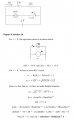# Second Order Circuit

#### Bernardri

Joined Dec 17, 2018
5
Hello, I would like some help with this second order circuit exercise.

I did'nt understand why the solution says that V (0) = -30 V.

Also, I did not understand why the capacitor's voltage (Vc) is -30 V.

Isn't the voltage for t <0 (when the capacitor is loaded) the same as the voltage source?

The exercise asked for the circuit below i(t) for t>0 . The switch opens at t=0 after a long time closedMy attempt:

For t<0: V(0)= 30 V and i(0)= 15 A

For t>0:

α= R/(2L)= 2/(2*0,5)= 2

omega = 1/sqrt (LC) = 1/sqrt(0.5*0.25) = 2.82942712475

Under-damped:

di/dt= -2(15 cos(2t)+ B sin(2t))e^-2t + (-2*15 sin (2t) + 2 Bcos(2t))e^2t

di(0)/dt = -30+ 2B = -(1/L) [R*i(o)+ Vc(0)] = -2 [30+30] = -120

-30+2B=-120

B= -45

i(t)= 15 cos (2t) + (-45 sin (2t))e^-2t A

So, the answer isn't the same as the book's solution.

#### Bernardri

Joined Dec 17, 2018
5
Apparently they are using the left side of the capacitor as the common/ground reference.
Below is the LTspice simulation of the circuit:
The response is slightly underdamped as you stated.

View attachment 166018
Do you think that the equation that describes i(t). i(t) = (15 cos (2t) + 15 sin(2t))e^-2t A , is correct?
Thank you very much, Crutshow.

#### crutschow

Joined Mar 14, 2008
30,098
Do you think that the equation that describes i(t). i(t) = (15 cos (2t) + 15 sin(2t))e^-2t A , is correct?
Not sure.
Sorry, I don't do math problems unless I absolutely have to.#### MrAl

Joined Jun 17, 2014
9,151
Hello, I would like some help with this second order circuit exercise.

I did'nt understand why the solution says that V (0) = -30 V.

Also, I did not understand why the capacitor's voltage (Vc) is -30 V.

Isn't the voltage for t <0 (when the capacitor is loaded) the same as the voltage source?

The exercise asked for the circuit below i(t) for t>0 . The switch opens at t=0 after a long time closed
View attachment 166014

My attempt:

For t<0: V(0)= 30 V and i(0)= 15 A

For t>0:

α= R/(2L)= 2/(2*0,5)= 2

omega = 1/sqrt (LC) = 1/sqrt(0.5*0.25) = 2.82942712475

Under-damped:

di/dt= -2(15 cos(2t)+ B sin(2t))e^-2t + (-2*15 sin (2t) + 2 Bcos(2t))e^2t

di(0)/dt = -30+ 2B = -(1/L) [R*i(o)+ Vc(0)] = -2 [30+30] = -120

-30+2B=-120

B= -45

i(t)= 15 cos (2t) + (-45 sin (2t))e^-2t A

So, the answer isn't the same as the book's solution.
Hello,

Are there some things missing from this problem statement?
I ask because the bottom of the second circuit diagram looks like it is missing.

Also, is the -30v the initial cap voltage? That's what it looks like, but it would be better if we could see the whole problem statement and also the solution too. This is not a difficult problem but we need the exact problem specification to answer adequately.